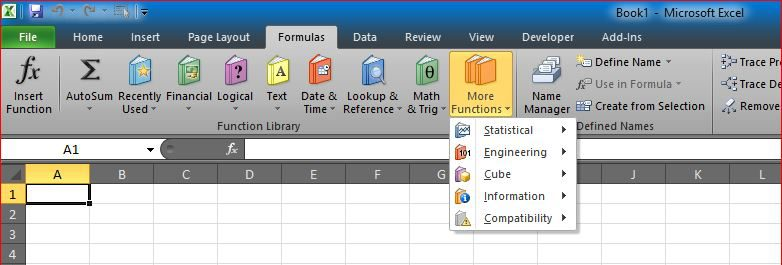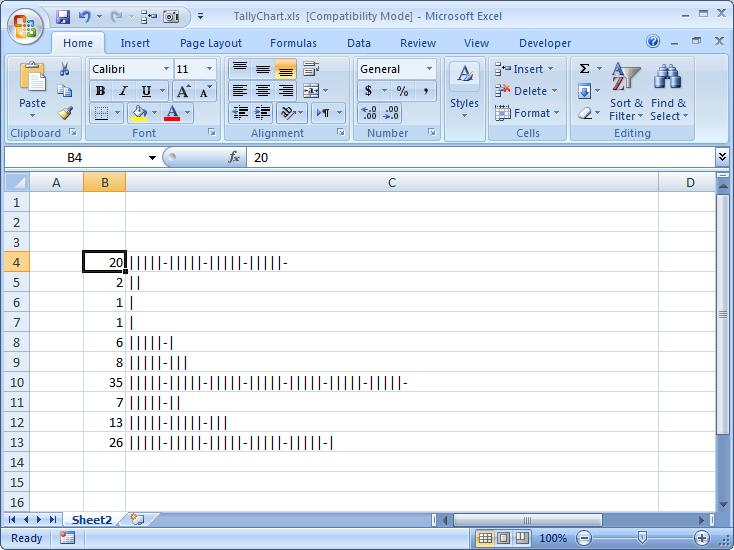# Tally chart in functionsUsing a single function can make a graphical view to your number

Function is REPT along with IF and INT to show that number in graphical view, formula used here is:

`=IF(B4<5,REPT("|",B4),REPT(REPT("|",5)&"-",INT(B4/5))&REPT("|",B4-INT(B4/5)*5))`